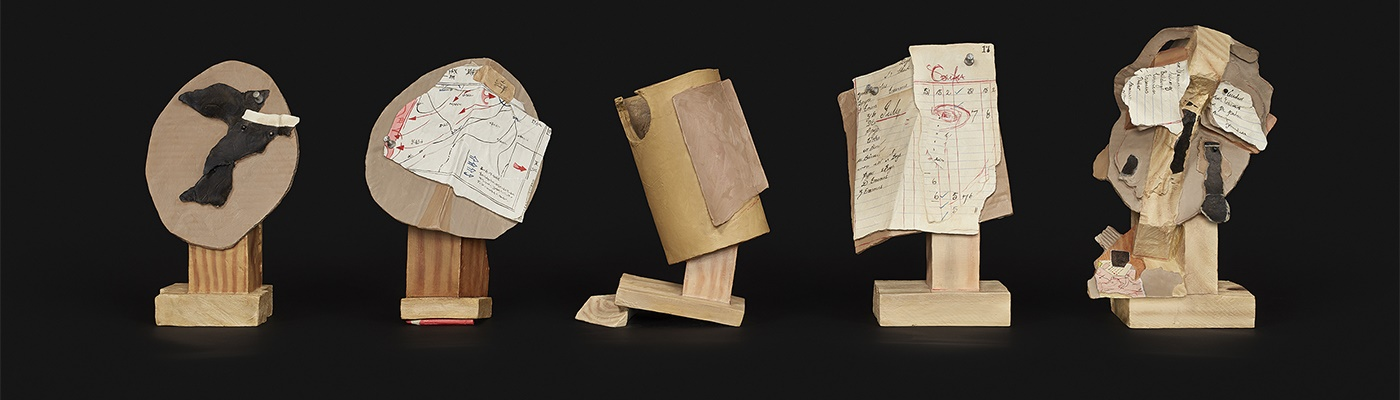##### Modern & Contemporary Art
Date: Sun, 1st Sep 2019 09:30
Location: Cape Town
Catalogue: View e-Catalogue

Name: Ms Emma Bedford
Email: emma@aspireart.net
Telephone: +27 83 391 7235

### Refine your results

Category

Sold for R34,140
Estimated at R20,000 - R30,000

Sold for R54,624
Estimated at R40,000 - R60,000

Sold for R147,940
Estimated at R80,000 - R120,000

Sold for R147,940
Estimated at R80,000 - R120,000

Sold for R125,180
Estimated at R100,000 - R150,000

Sold for R56,900
Estimated at R50,000 - R70,000

Estimated at R90,000 - R120,000

Sold for R398,300
Estimated at R500,000 - R800,000

Sold for R295,880
Estimated at R220,000 - R280,000

Estimated at R80,000 - R120,000

Estimated at R60,000 - R90,000

Sold for R295,880
Estimated at R250,000 - R300,000

Sold for R27,312
Estimated at R30,000 - R50,000

Estimated at R140,000 - R180,000

Sold for R227,600
Estimated at R150,000 - R250,000

Sold for R682,800
Estimated at R600,000 - R900,000

Sold for R3,657,857
Estimated at R4,000,000 - R6,000,000

Sold for R2,731,200
Estimated at R2,200,000 - R3,200,000

Sold for R170,700
Estimated at R100,000 - R150,000

Sold for R52,348
Estimated at R25,000 - R35,000

Sold for R170,700
Estimated at R150,000 - R200,000

Sold for R193,460
Estimated at R140,000 - R180,000

Sold for R43,244
Estimated at R30,000 - R40,000

Estimated at R40,000 - R60,000

Estimated at R70,000 - R100,000

Estimated at R50,000 - R80,000

Sold for R34,140
Estimated at R30,000 - R40,000

Sold for R318,640
Estimated at R150,000 - R250,000

Sold for R170,700
Estimated at R150,000 - R250,000

Sold for R51,210
Estimated at R50,000 - R70,000

Sold for R68,280
Estimated at R25,000 - R30,000

Sold for R21,105
Estimated at R18,000 - R24,000

Sold for R136,560
Estimated at R90,000 - R120,000

Estimated at R40,000 - R60,000

Sold for R36,416
Estimated at R40,000 - R60,000

Sold for R45,520
Estimated at R30,000 - R50,000

Sold for R193,460
Estimated at R100,000 - R150,000

Sold for R25,036
Estimated at R15,000 - R20,000

Estimated at R20,000 - R30,000

Estimated at R20,000 - R30,000

Sold for R39,830
Estimated at R50,000 - R80,000

Estimated at R90,000 - R120,000

Sold for R56,900
Estimated at R50,000 - R70,000

Sold for R50,072
Estimated at R50,000 - R70,000

Sold for R113,800
Estimated at R80,000 - R120,000

Estimated at R25,000 - R35,000

Sold for R34,140
Estimated at R30,000 - R40,000

Sold for R45,520
Estimated at R40,000 - R60,000